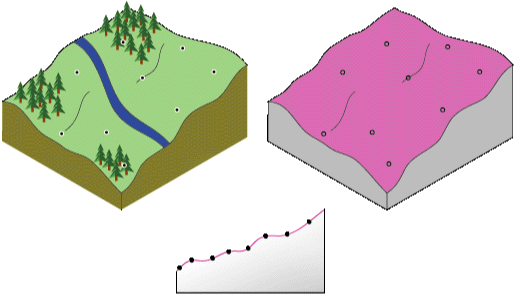# How radial basis functions work

Mit der Geostatistical Analyst-Lizenz verfügbar.

Radial basis functions (RBFs) are a series of exact interpolation techniques; that is, the surface must pass through each measured sample value. There are five different basis functions:

• Thin-plate spline
• Spline with tension
• Completely regularized spline

Each basis function has a different shape and results in a different interpolation surface. RBF methods are a special case of splines.

RBFs are conceptually similar to fitting a rubber membrane through the measured sample values while minimizing the total curvature of the surface. The basis function you select determines how the rubber membrane will fit between the values. The following diagram illustrates conceptually how an RBF surface fits through a series of elevation sample values. Notice in the cross section, the surface passes through the data values.Cross section illustrating how RBF surface fits through the sample values

Being exact interpolators, the RBF methods differ from the global and local polynomial interpolators, which are both inexact interpolators that do not require the surface to pass through the measured points. When comparing an RBF to IDW (which is also an exact interpolator), IDW will never predict values above the maximum measured value or below the minimum measured value as you can see in the cross section of a transect of sample data below.

However, the RBFs can predict values above the maximum and below the minimum measured values as in the cross section below.

Optimal parameters are determined using cross validation in a similar manner as explained for IDW and local polynomial interpolation.

## When to use radial basis functions

RBFs are used to produce smooth surfaces from a large number of data points. The functions produce good results for gently varying surfaces such as elevation.

However, the techniques are inappropriate when large changes in the surface values occur within short distances and/or when you suspect the sample data is prone to measurement error or uncertainty.

## Concepts behind radial basis functions

In Geostatistical Analyst, RBFs are formed over each data location. An RBF is a function that changes with distance from a location.